# Class 10 Maths Chapter 10 Circles

NCERT Solutions

NCERT Solutions for Class 10 Maths Chapter 10 Circles

Page No: 209

Exercise: 10.1

1. How many tangents can a circle have?

A circle can have infinite tangents.

2.  Fill in the blanks :
(i) A tangent to a circle intersects it in ............... point(s).
(ii) A line intersecting a circle in two points is called a .............
(iii) A circle can have ............... parallel tangents at the most.
(iv) The common point of a tangent to a circle and the circle is called ............

(i) one
(ii) secant
(iii) two
(iv) point of contact

3. A tangent PQ at a point P of a circle of radius 5 cm meets a line through the centre O at
a point Q so that OQ = 12 cm. Length PQ is :
(A) 12 cm
(B) 13 cm
(C) 8.5 cm
(D) √119 cmThe line drawn from the centre of the circle to the tangent is perpendicular to the tangent.
∴ OP ⊥ PQ
By Pythagoras theorem in ΔOPQ,
OQ2 = OP2 + PQ2
⇒ (12)= 52 + PQ2
⇒PQ2 = 144 - 25
⇒PQ2 = 119
⇒PQ = √119 cm
(D) is the correct option.

4. Draw a circle and two lines parallel to a given line such that one is a tangent and the
other, a secant to the circle.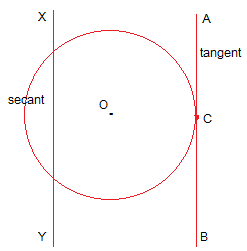AB and XY are two parallel lines where AB is the tangent to the circle at point C while XY is the secant to the circle.

Page NO: 213

Exercise: 10.2

In Q.1 to 3, choose the correct option and give justification.

1.  From a point Q, the length of the tangent to a circle is 24 cm and the distance of Q from the centre is 25 cm. The radius of the circle is
(A)  7 cm
(B) 12 cm
(C) 15 cm
(D) 24.5 cm

The line drawn from the centre of the circle to the tangent is perpendicular to the tangent.∴ OP ⊥ PQ
also, ΔOPQ is right angled.
OQ = 25 cm and PQ = 24 cm (Given)
By Pythagoras theorem in ΔOPQ,
OQ2 = OP2 + PQ2
⇒ (25)= OP2 + (24)2
⇒ OP2 = 625 - 576
⇒ OP2 = 49
⇒ OP = 7 cm
The radius of the circle is option (A) 7 cm.

2.  In Fig. 10.11, if TP and TQ are the two tangents to a circle with centre O so that ∠POQ = 110°, then ∠PTQ is equal to
(A) 60°
(B) 70°
(C) 80°
(D) 90°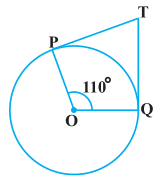OP and OQ are radii of the circle to the tangents TP and TQ respectively.
∴ OP ⊥ TP and,
∴ OQ ⊥ TQ
∠OPT = ∠OQT = 90°
Sum of all interior angles = 360°
∠PTQ + ∠OPT + ∠POQ + ∠OQT  = 360°
⇒ ∠PTQ + 90° + 110° + 90°  = 360°
⇒ ∠PTQ = 70°
∠PTQ is equal to option (B) 70°.

3.  If tangents PA and PB from a point P to a circle with centre O are inclined to each other at angle of 80°, then ∠ POA is equal to
(A) 50°
(B) 60°
(C) 70°
(D) 80°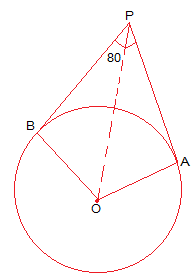OA and OB are radii of the circle to the tangents PA and PB respectively.
∴ OA ⊥ PA and,
∴ OB ⊥ PB
∠OBP = ∠OAP = 90°
Sum of all interior angles = 360°
∠AOB + ∠OBP + ∠OAP + ∠APB  = 360°
⇒ ∠AOB + 90° + 90° + 80°  = 360°
⇒ ∠AOB = 100°
Now,
In ΔOPB and ΔOPA,
AP = BP (Tangents from a point are equal)
OA = OB (Radii of the circle)
OP = OP (Common side)
∴ ΔOPB ≅ ΔOPA (by SSS congruence condition)
Thus ∠POB = ∠POA
∠AOB = ∠POB + ∠POA
⇒ 2 ∠POA = ∠AOB
⇒ ∠POA = 100°/2 = 50°
∠POA is equal to option  (A) 50°

Page No: 214

4.  Prove that the tangents drawn at the ends of a diameter of a circle are parallel.

Let AB be a diameter of the circle. Two tangents PQ and RS are drawn at points A and B respectively.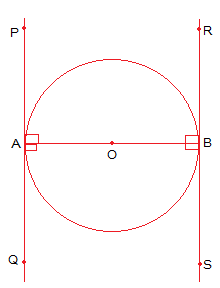Radii of the circle to the tangents will be perpendicular to it.
∴ OB ⊥ RS and,
∴ OA ⊥ PQ
∠OBR = ∠OBS = ∠OAP = ∠OAQ = 90º
From the figure,
∠OBR = ∠OAQ (Alternate interior angles)
∠OBS = ∠OAP (Alternate interior angles)
Since alternate interior angles are equal, lines PQ and RS will be parallel.
Hence Proved that the tangents drawn at the ends of a diameter of a circle are parallel.

5.  Prove that the perpendicular at the point of contact to the tangent to a circle passes through the centre.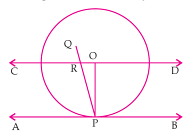Let AB be the tangent to the circle at point P with centre O.
We have to prove that PQ passes through the point O.
Suppose that PQ doesn't passes through point O. Join OP.
Through O, draw a straight line CD parallel to the tangent AB.
PQ intersect CD at R and also intersect AB at P.
AS, CD // AB PQ is the line of intersection,
∠ORP = ∠RPA (Alternate interior angles)
but also,
∠RPA = 90° (PQ ⊥ AB)
⇒ ∠ORP  = 90°
∠ROP + ∠OPA = 180° (Co-interior angles)
⇒∠ROP + 90° = 180°
⇒∠ROP = 90°
Thus, the ΔORP has 2 right angles i.e. ∠ORP  and ∠ROP which is not possible.
Hence, our supposition is wrong.
∴ PQ passes through the point O.

6.  The length of a tangent from a point A at distance 5 cm from the centre of the circle is 4 cm. Find the radius of the circle.

AB is a tangent drawn on this circle from point A.∴ OB ⊥ AB
OA = 5cm and AB = 4 cm (Given)
In ΔABO,
By Pythagoras theorem in ΔABO,
OA2 = AB+ BO2
⇒ 5= 4+ BO2
⇒ BO2 = 25 - 16
⇒ BO2 = 9
⇒ BO = 3
∴ The radius of the circle is 3 cm.

7. Two concentric circles are of radii 5 cm and 3 cm. Find the length of the chord of the
larger circle which touches the smaller circle.Let the two concentric circles with centre O.
AB be the chord of the larger circle which touches the smaller circle at point P.
∴ AB is tangent to the smaller circle to the point P.
⇒ OP ⊥ AB
By Pythagoras theorem in ΔOPA,
OA2 =  AP2 + OP2
⇒ 52 = AP2 + 32
⇒ AP2 = 25 - 9
⇒ AP = 4
In ΔOPB,
Since OP ⊥ AB,
AP = PB (Perpendicular from the center of the circle bisects the chord)
AB = 2AP = 2 × 4 = 8 cm
∴ The length of the chord of the larger circle is 8 cm.

8. A quadrilateral ABCD is drawn to circumscribe a circle (see Fig. 10.12). Prove that AB + CD = AD + BCFrom the figure we observe that,
DR = DS (Tangents on the circle from point D) … (i)
AP = AS (Tangents on the circle from point A) … (ii)
BP = BQ (Tangents on the circle from point B) … (iii)
CR = CQ (Tangents on the circle from point C) … (iv)
DR + AP + BP + CR = DS + AS + BQ + CQ
⇒ (BP + AP) + (DR + CR)  = (DS + AS) + (CQ + BQ)
⇒ CD + AB = AD + BC

9. In Fig. 10.13, XY and X′Y′ are two parallel tangents to a circle with centre O and another tangent AB with point of contact C intersecting XY at A and X′Y′ at B. Prove that ∠ AOB = 90°.

We joined O and C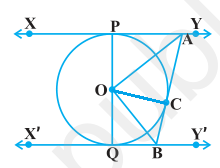A/q,
In ΔOPA and ΔOCA,
OP = OC (Radii of the same circle)
AP = AC (Tangents from point A)
AO = AO (Common side)
∴ ΔOPA ≅ ΔOCA (SSS congruence criterion)
⇒ ∠POA = ∠COA … (i)
Similarly,
ΔOQB  ≅ ΔOCB
∠QOB = ∠COB … (ii)
Since POQ is a diameter of the circle, it is a straight line.
∴ ∠POA + ∠COA + ∠COB + ∠QOB = 180 º
From equations (i) and (ii),
2∠COA + 2∠COB = 180º
⇒ ∠COA + ∠COB = 90º
⇒ ∠AOB = 90°

10.  Prove that the angle between the two tangents drawn from an external point to a circle is supplementary to the angle subtended by the line-segment joining the points of contact at the centre.Consider a circle with centre O. Let P be an external point from which two tangents PA and PB are drawn to the circle which are touching the circle at point A and B respectively and AB is the line segment, joining point of contacts A and B together such that it subtends ∠AOB at center O of the circle.
It can be observed that
OA ⊥ PA
∴ ∠OAP = 90°
Similarly, OB ⊥ PB
∴ ∠OBP = 90°
Sum of all interior angles = 360º
∠OAP +∠APB +∠PBO +∠BOA = 360º
⇒ 90º + ∠APB + 90º + ∠BOA = 360º
⇒ ∠APB + ∠BOA = 180º
∴ The angle between the two tangents drawn from an external point to a circle is supplementary to the angle subtended by the line-segment joining the points of contact at the centre.

11. Prove that the parallelogram circumscribing a circle is a rhombus.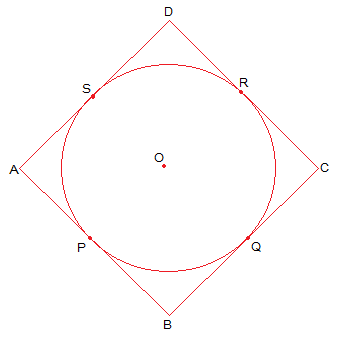ABCD is a parallelogram,
∴ AB = CD ... (i)
∴ BC = AD ... (ii)
From the figure, we observe that,
DR = DS (Tangents to the circle at D)
CR = CQ (Tangents to the circle at C)
BP = BQ (Tangents to the circle at B)
AP = AS (Tangents to the circle at A)
DR + CR + BP + AP = DS + CQ + BQ + AS
⇒ (DR + CR) + (BP + AP) = (DS + AS) + (CQ + BQ)
⇒ CD + AB = AD + BC ... (iii)
Putting the value of (i) and (ii) in equation (iii) we get,
2AB = 2BC
⇒ AB = BC ... (iv)
By Comparing equations (i), (ii), and (iv) we get,
AB = BC = CD = DA
∴ ABCD is a rhombus.

12. A triangle ABC is drawn to circumscribe a circle of radius 4 cm such that the segments BD and DC into which BC is divided by the point of contact D are of lengths 8 cm and 6 cm respectively (see Fig. 10.14). Find the sides AB and AC.In ΔABC,
Length of two tangents drawn from the same point to the circle are equal,
∴ CF = CD = 6cm
∴ BE = BD = 8cm
∴ AE = AF = x
We observed that,
AB = AE + EB = x + 8
BC = BD + DC = 8 + 6 = 14
CA = CF + FA = 6 + x
Now semi perimeter of triangle (s) is,
⇒ 2s = AB + BC + CA
x + 8 + 14 + 6 + x
= 28 + 2x
⇒s = 14 + x
Area of ΔABC = √s (s - a)(s - b)(s - c)
= √(14 + x) (14 + - 14)(14 + - x - 6)(14 + - x - 8)
= √(14 + x) (x)(8)(6)
= √(14 + x) 48 x ... (i)
also, Area of ΔABC = 2×area of (ΔAOF + ΔCOD + ΔDOB)
= 2×[(1/2×OF×AF) + (1/2×CD×OD) + (1/2×DB×OD)]
= 2×1/2 (4+ 24 + 32) = 56 + 4... (ii)
Equating equation (i) and (ii) we get,
(14 + x) 48 = 56 + 4x
Squaring both sides,
48x (14 + x) = (56 + 4x)2
⇒ 48x = [4(14 + x)]2/(14 + x)
⇒ 48x = 16 (14 + x)
⇒ 48x = 224 + 16x
⇒ 32x = 224
⇒ x = 7 cm
Hence, AB = x + 8 = 7 + 8 = 15 cm
CA = 6 + x = 6 + 7 = 13 cm

13.  Prove that opposite sides of a quadrilateral circumscribing a circle subtend supplementary angles at the centre of the circle.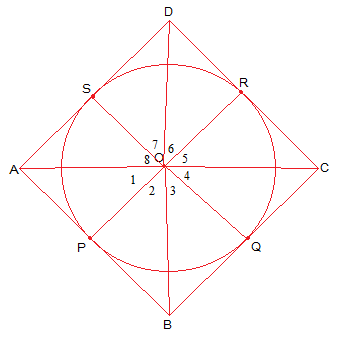Let ABCD be a quadrilateral circumscribing a circle with O such that it touches the circle at point P, Q, R, S. Join the vertices of the quadrilateral ABCD to the center of the circle.
In ΔOAP and ΔOAS,
AP = AS (Tangents from the same point)
OP = OS (Radii of the circle)
OA = OA (Common side)
ΔOAP ≅ ΔOAS (SSS congruence condition)
∴ ∠POA = ∠AOS
⇒∠1 = ∠8
Similarly we get,
∠2 = ∠3
∠4 = ∠5
∠6 = ∠7